Normal Distribution Notes The normal distribution is considered

• Slides: 39Normal Distribution • • • • • • • • •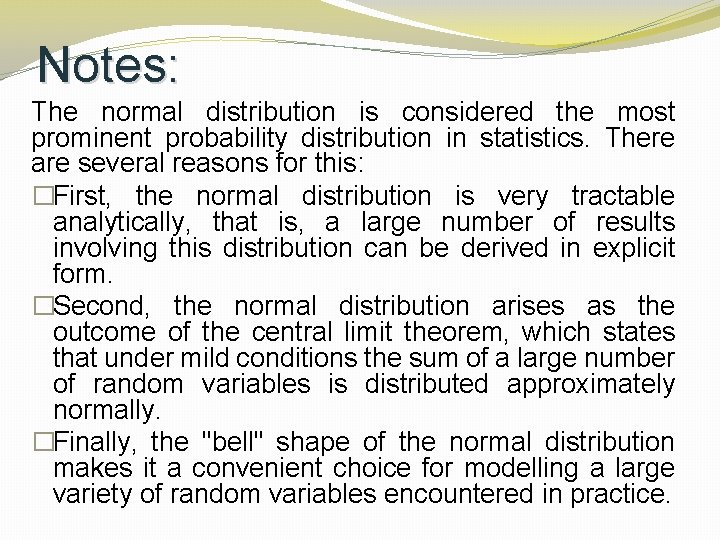Notes: The normal distribution is considered the most prominent probability distribution in statistics. There are several reasons for this: �First, the normal distribution is very tractable analytically, that is, a large number of results involving this distribution can be derived in explicit form. �Second, the normal distribution arises as the outcome of the central limit theorem, which states that under mild conditions the sum of a large number of random variables is distributed approximately normally. �Finally, the "bell" shape of the normal distribution makes it a convenient choice for modelling a large variety of random variables encountered in practice.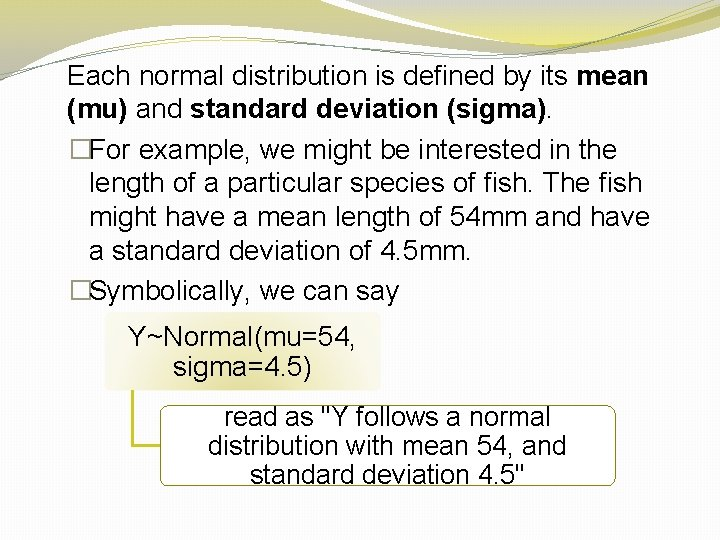Each normal distribution is defined by its mean (mu) and standard deviation (sigma). �For example, we might be interested in the length of a particular species of fish. The fish might have a mean length of 54 mm and have a standard deviation of 4. 5 mm. �Symbolically, we can say Y~Normal(mu=54, sigma=4. 5) read as "Y follows a normal distribution with mean 54, and standard deviation 4. 5"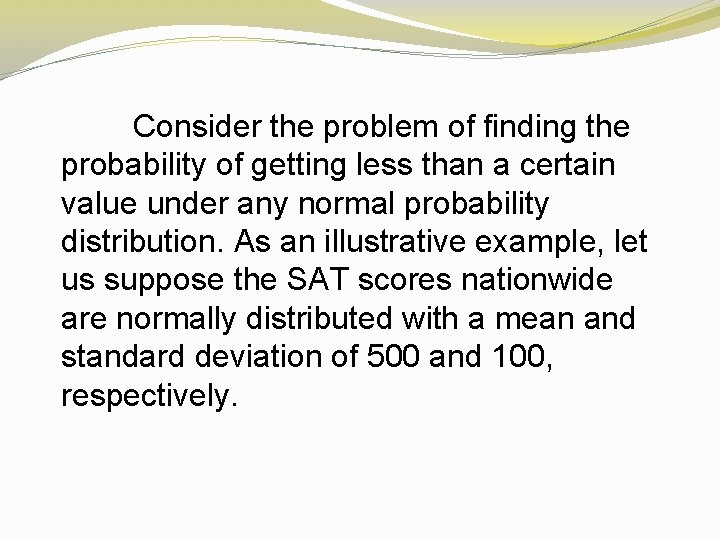Consider the problem of finding the probability of getting less than a certain value under any normal probability distribution. As an illustrative example, let us suppose the SAT scores nationwide are normally distributed with a mean and standard deviation of 500 and 100, respectively.Answer the following questions based on the given information: A. What is the probability that a randomly selected student score will be less than 600 points? B. What is the probability that a randomly selected student score will exceed 600 points? C. What is the probability that a randomly selected student score will be between 400 and 600?Hint: Using Excel you can find the probability of getting a value approximately less than or equal to a given value. In a problem, when the mean and the standard deviation of the population are given, you have to use common sense to find different probabilities based on the question since you know the area under a normal curve is 1.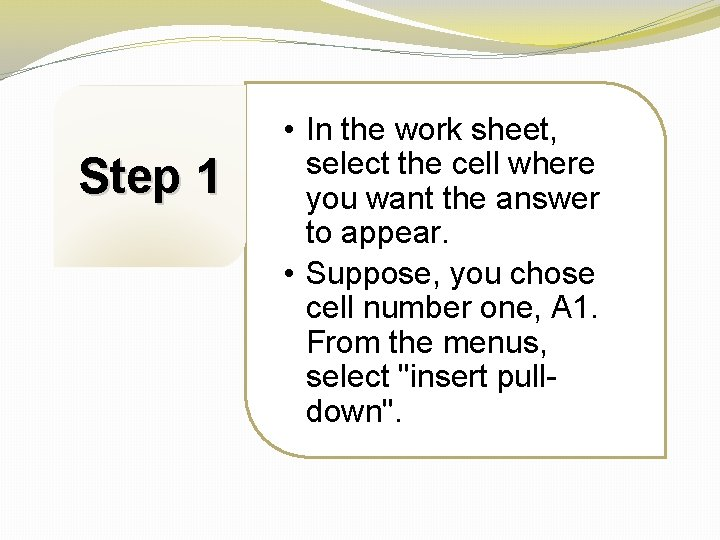Step 1 • In the work sheet, select the cell where you want the answer to appear. • Suppose, you chose cell number one, A 1. From the menus, select "insert pulldown".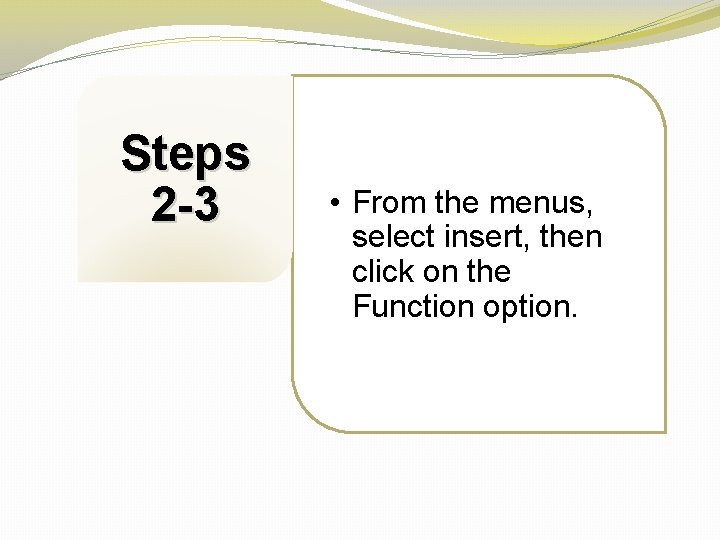Steps 2 -3 • From the menus, select insert, then click on the Function option.Step 4 • After clicking on the Function option, the Paste Function dialog appears from Function Category. Choose Statistical then NORMDIST from the Function Name box. Click OK.Step 5 • After clicking on OK, the NORMDIST distribution box appears: i. Enter 600 in X (the value box) ii. Enter 500 in the Mean box iii. Enter 100 in the Standard deviation box iv. Type "true" in the cumulative box, then click OK.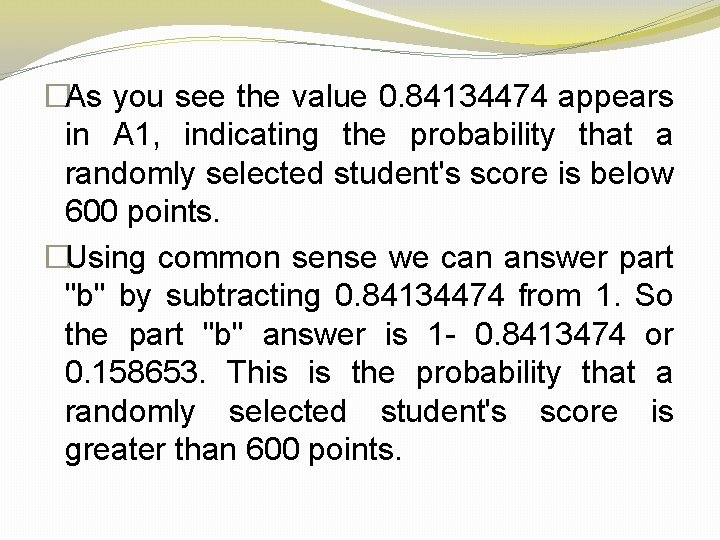�As you see the value 0. 84134474 appears in A 1, indicating the probability that a randomly selected student's score is below 600 points. �Using common sense we can answer part "b" by subtracting 0. 84134474 from 1. So the part "b" answer is 1 - 0. 8413474 or 0. 158653. This is the probability that a randomly selected student's score is greater than 600 points.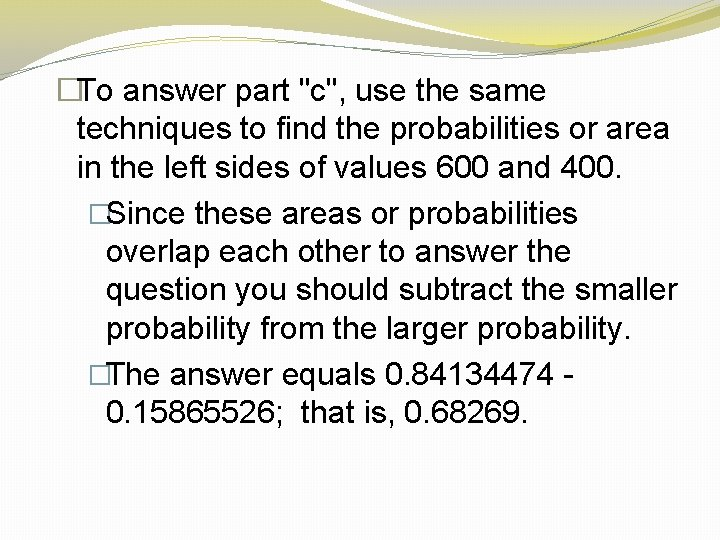�To answer part "c", use the same techniques to find the probabilities or area in the left sides of values 600 and 400. �Since these areas or probabilities overlap each other to answer the question you should subtract the smaller probability from the larger probability. �The answer equals 0. 84134474 - 0. 15865526; that is, 0. 68269.Inverse Case • Calculating the value of a random variable often called the "x" value�You can use NORMINV from the function box to calculate a value for the random variable - if the probability to the left side of this variable is given. Actually, you should use this function to calculate different percentiles. �In this problem one could ask what is the score of a student whose percentile is 90? This means approximately 90% of students scores are less than this number. �On the other hand if we were asked to do this problem by hand, we would have had to calculate the x value using the normal distribution formula x = m + zd.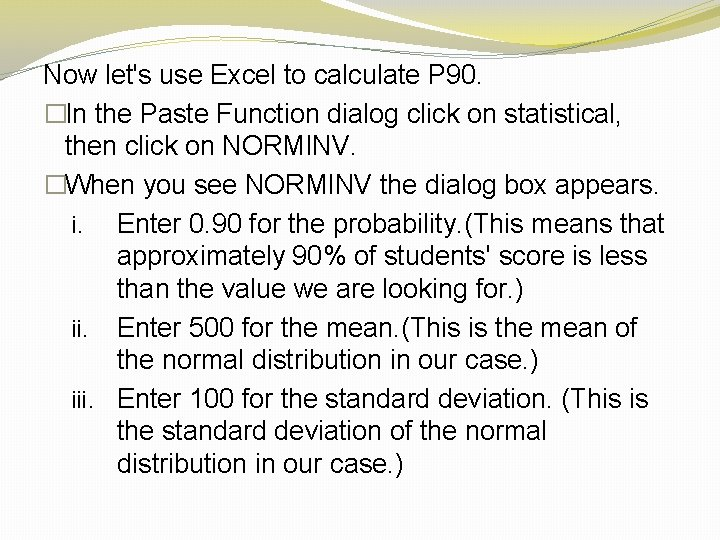Now let's use Excel to calculate P 90. �In the Paste Function dialog click on statistical, then click on NORMINV. �When you see NORMINV the dialog box appears. i. Enter 0. 90 for the probability. (This means that approximately 90% of students' score is less than the value we are looking for. ) ii. Enter 500 for the mean. (This is the mean of the normal distribution in our case. ) iii. Enter 100 for the standard deviation. (This is the standard deviation of the normal distribution in our case. )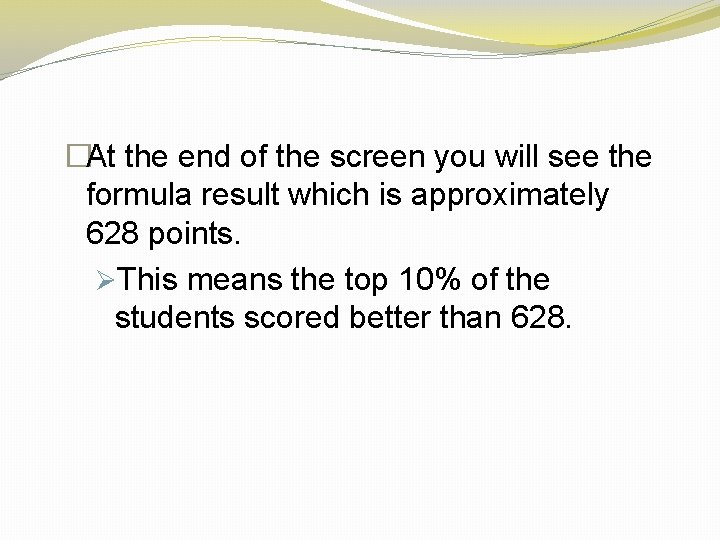�At the end of the screen you will see the formula result which is approximately 628 points. ØThis means the top 10% of the students scored better than 628.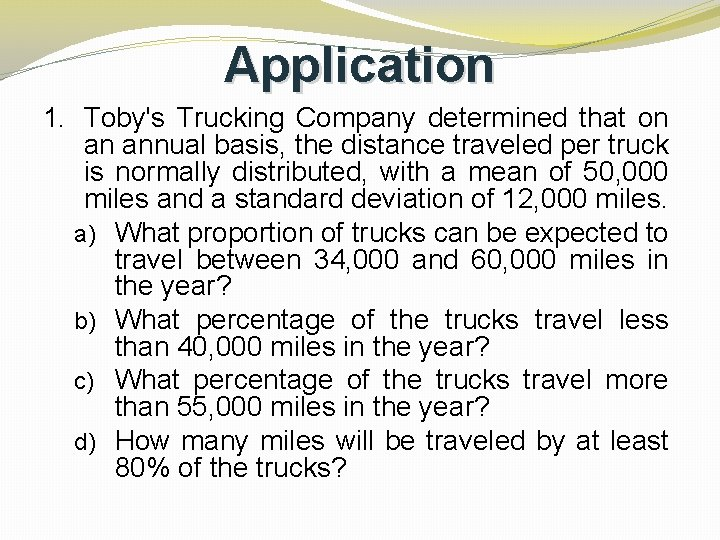Application 1. Toby's Trucking Company determined that on an annual basis, the distance traveled per truck is normally distributed, with a mean of 50, 000 miles and a standard deviation of 12, 000 miles. a) What proportion of trucks can be expected to travel between 34, 000 and 60, 000 miles in the year? b) What percentage of the trucks travel less than 40, 000 miles in the year? c) What percentage of the trucks travel more than 55, 000 miles in the year? d) How many miles will be traveled by at least 80% of the trucks?For A: In Excel Insert 2010, click Formulas You should see a box like this: Type in NORMDIST and select.�To find the probability of traveling between 34, 000 and 60, 000, ØFind the probability of 60, 000 and then subtract from the probability of 34, 000 �To find the probability of 60, 000, ØInput 60, 000 for X; 50, 000 for MEAN; 12, 000 for Standard Division; and TRUE for Cumulative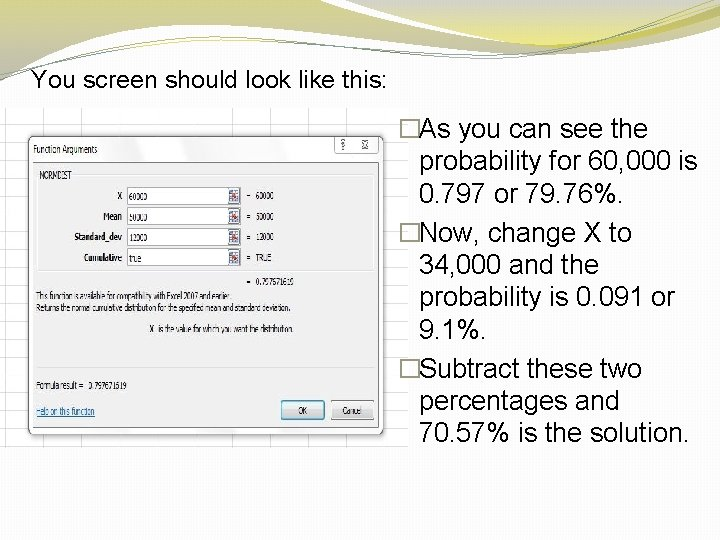You screen should look like this: �As you can see the probability for 60, 000 is 0. 797 or 79. 76%. �Now, change X to 34, 000 and the probability is 0. 091 or 9. 1%. �Subtract these two percentages and 70. 57% is the solution.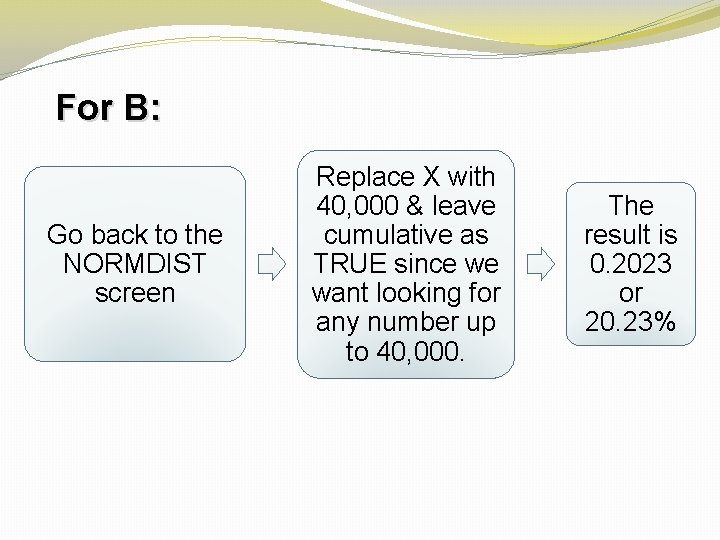For B: Go back to the NORMDIST screen Replace X with 40, 000 & leave cumulative as TRUE since we want looking for any number up to 40, 000. The result is 0. 2023 or 20. 23%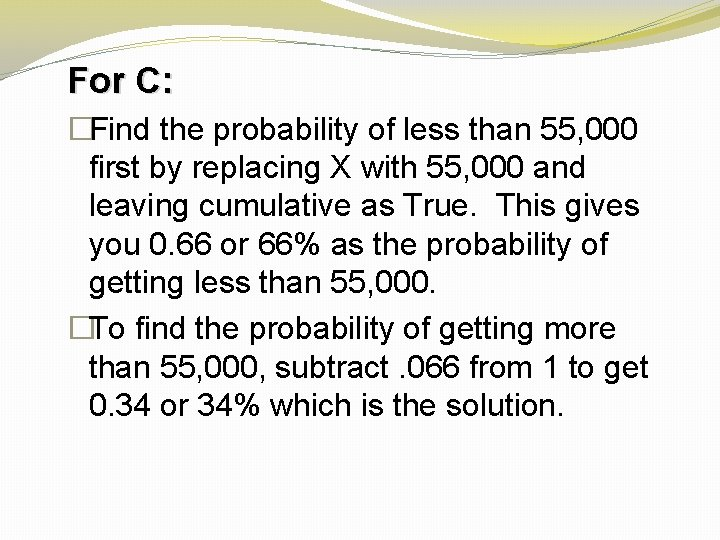For C: �Find the probability of less than 55, 000 first by replacing X with 55, 000 and leaving cumulative as True. This gives you 0. 66 or 66% as the probability of getting less than 55, 000. �To find the probability of getting more than 55, 000, subtract. 066 from 1 to get 0. 34 or 34% which is the solution.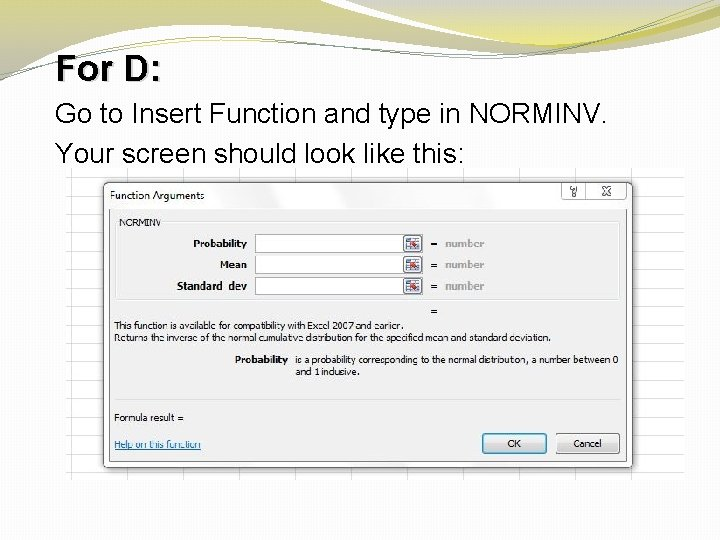For D: Go to Insert Function and type in NORMINV. Your screen should look like this: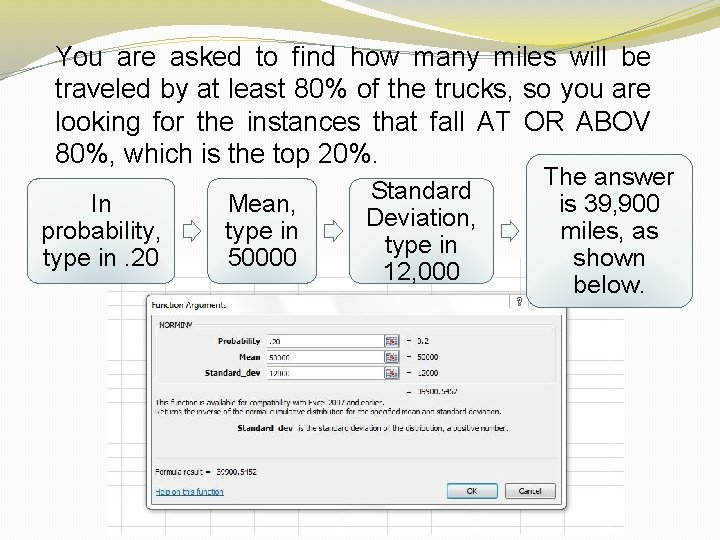You are asked to find how many miles will be traveled by at least 80% of the trucks, so you are looking for the instances that fall AT OR ABOV 80%, which is the top 20%. In probability, type in. 20 Mean, type in 50000 Standard Deviation, type in 12, 000 The answer is 39, 900 miles, as shown below.Application 2. Plastic bags used for packaging produce are manufactured so that the breaking strength of the bag is normally distributed with a mean of 5 pounds per square inch (psi) and a standard deviation of 1. 5 psi. a) What proportion of the bags produced have a breaking strength between 3. 2 and 4. 2 psi? b) What proportion of the bags produced have a breaking strength of at least 3. 6 psi? c) What proportion of the bags produced have a breaking strength of less than 6 psi? d) 30% of the bags are weaker than what breaking strength?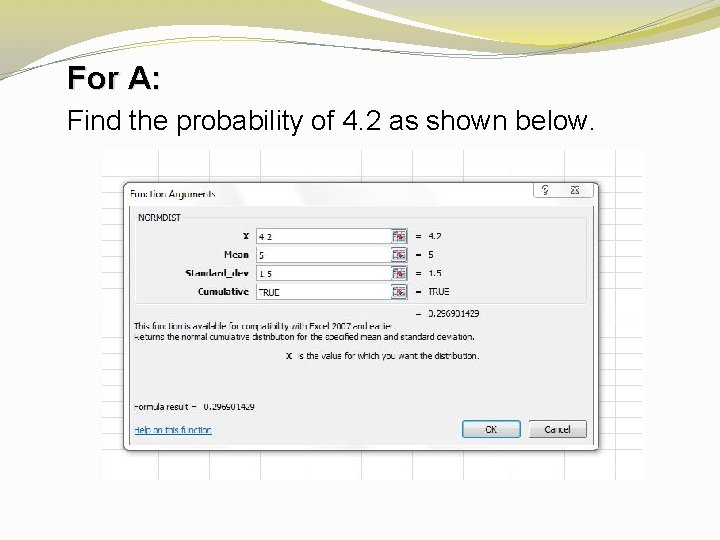For A: Find the probability of 4. 2 as shown below.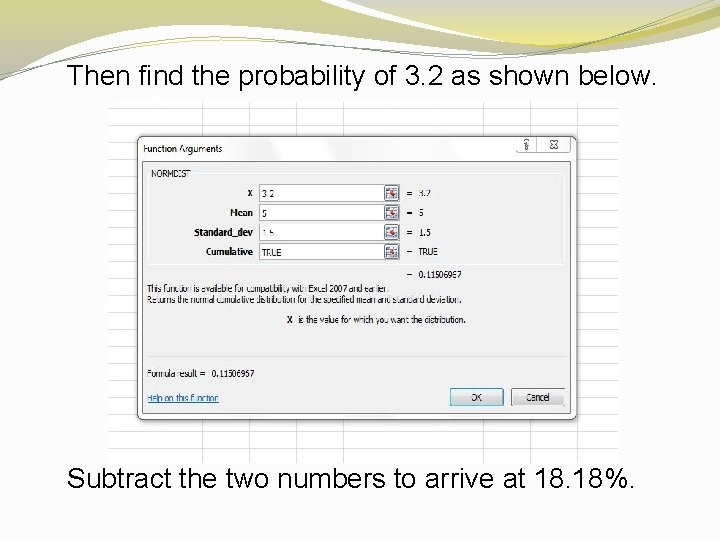Then find the probability of 3. 2 as shown below. Subtract the two numbers to arrive at 18. 18%.For B: Since we are looking for at least 3. 6 subtract the result from 1 to get 82. 46%.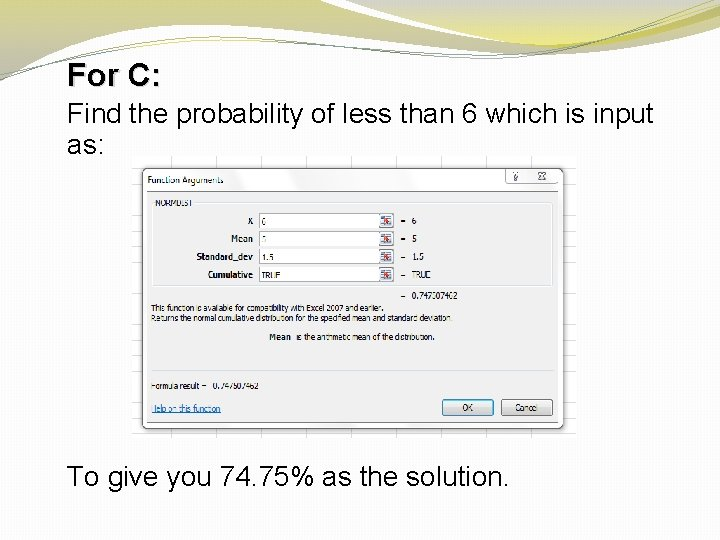For C: Find the probability of less than 6 which is input as: To give you 74. 75% as the solution.For D: Use NORMINV. Since we are looking at less than 30% we will use. 30 as the probability as shown below: Which gives us our solution of 4. 21 psi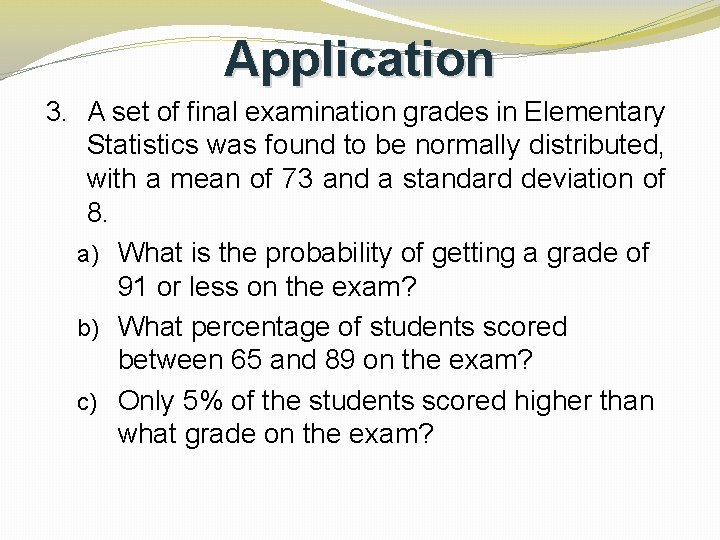Application 3. A set of final examination grades in Elementary Statistics was found to be normally distributed, with a mean of 73 and a standard deviation of 8. a) What is the probability of getting a grade of 91 or less on the exam? b) What percentage of students scored between 65 and 89 on the exam? c) Only 5% of the students scored higher than what grade on the exam?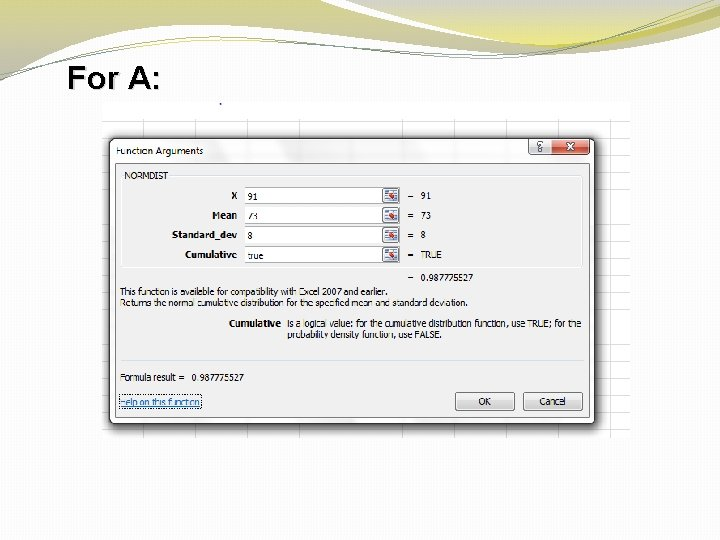For A: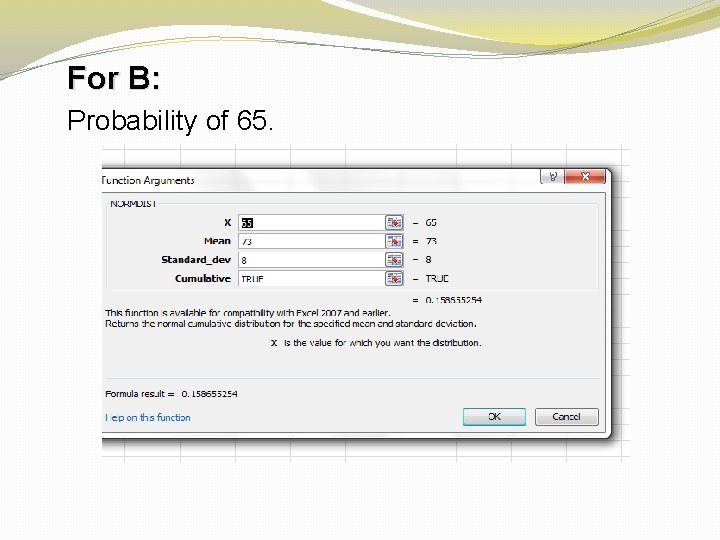For B: Probability of 65.Find the probability of 89. Now subtract the two to arrive at the solution of 81. 85%.For C: We use the probability of above 5%, which gives us what 95% of the students’ scores, and we can assume the remaining 5% score HIGHER than this.Application 4. A statistical analysis of 1, 000 long distance phone calls made from City Hall indicates that the length of these calls is normally distributed with a mean of 240 seconds and a standard deviation of 40 seconds. a) What percentage of these calls lasted less than 180 seconds? b) What is the probability that a particular call lasted between 180 and 300 seconds? c) What is the length of a particular call if only 1% of all calls are shorter?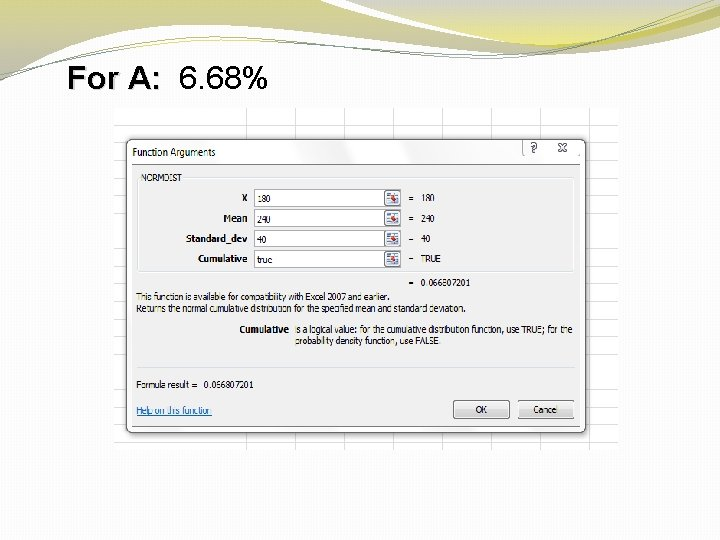For A: 6. 68%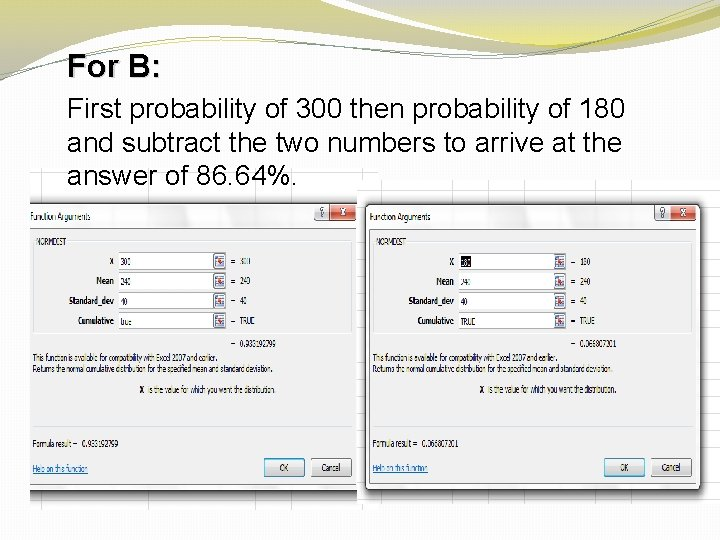For B: First probability of 300 then probability of 180 and subtract the two numbers to arrive at the answer of 86. 64%.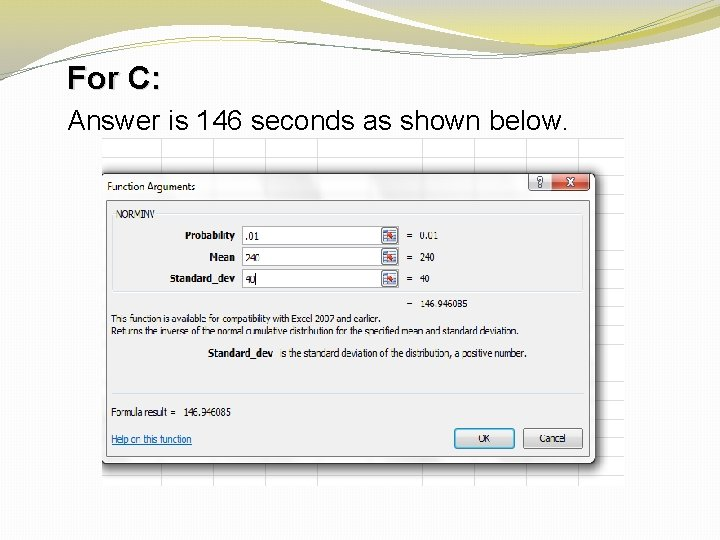For C: Answer is 146 seconds as shown below.